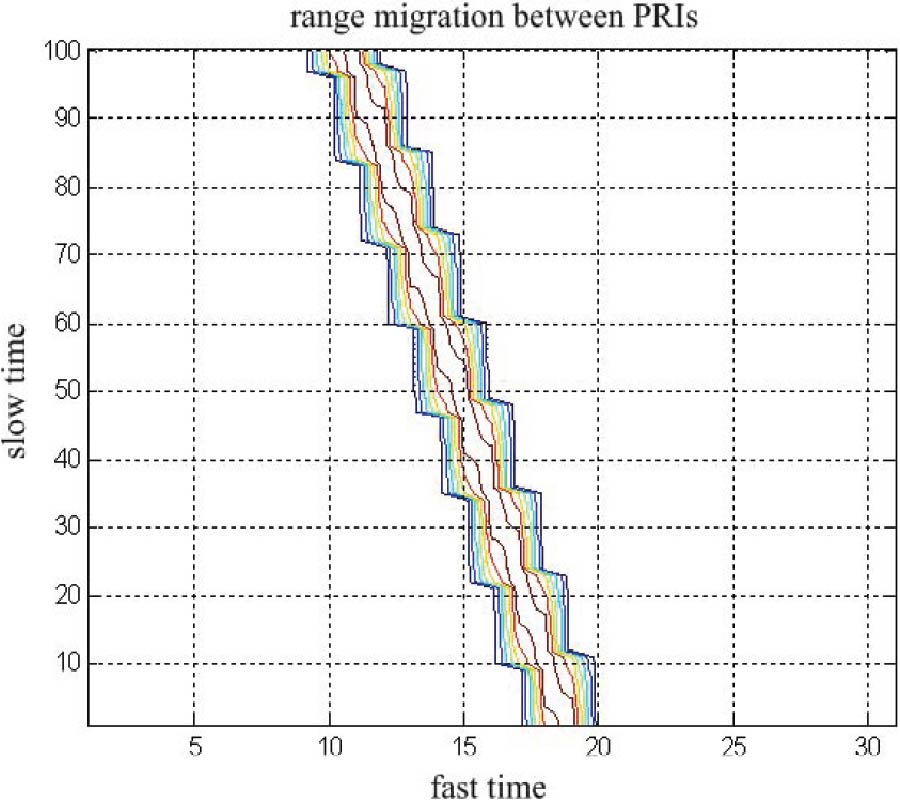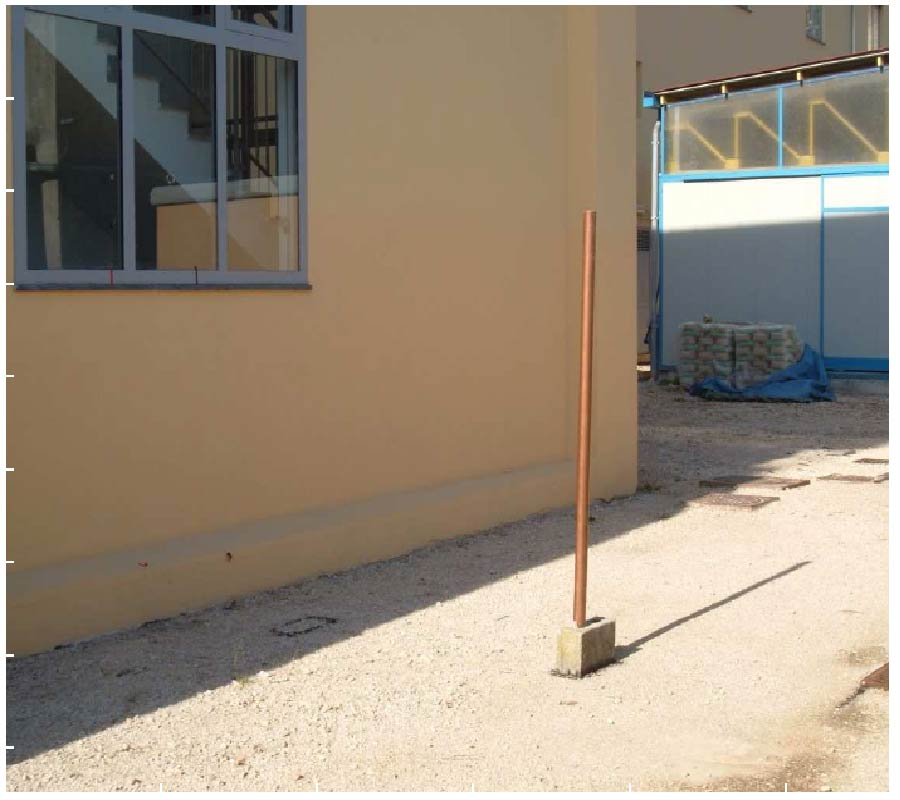Vol. 7
Latest Volume
All Volumes
All Journals
2009-07-02
PIER M
Vol. 7, 193-205, 2009
Non-Uniqueness of T-Charts for Solving Ccitl Problems with Passive Characteristic Impedances
Kanokwan Vudhivorn , Danai Torrungrueng and Chananya Thimaporn
Conjugately characteristic-impedance transmission lines (CCITLs) implemented by lossless periodic transmission-line structures have found various applications in microwave technology, and the T-chart was developed to perform the analysis and design of CCITLs effectively. Originally, the normalization factor used in defining normalized impedances of the T-chart is the geometric mean of characteristic impedances of CCITLs, which is not only one possible choice. By using other normalization factors based on characteristic impedances, different graphical representations can be obtained; i.e., T-charts for CCITLs with passive characteristic impedances are not unique, and it depends on the associated normalization factor. In this study, three more possible normalization factors related to characteristic impedances of CCITLs are investigated. It is found that all T-charts for each normalization factor are strongly dependent on the argument of characteristic impedances of CCITLs in a complicated fashion. The original T-chart based on the geometric mean of characteristic impedances is found to be the most convenient graphical representation for solving CCITL problems.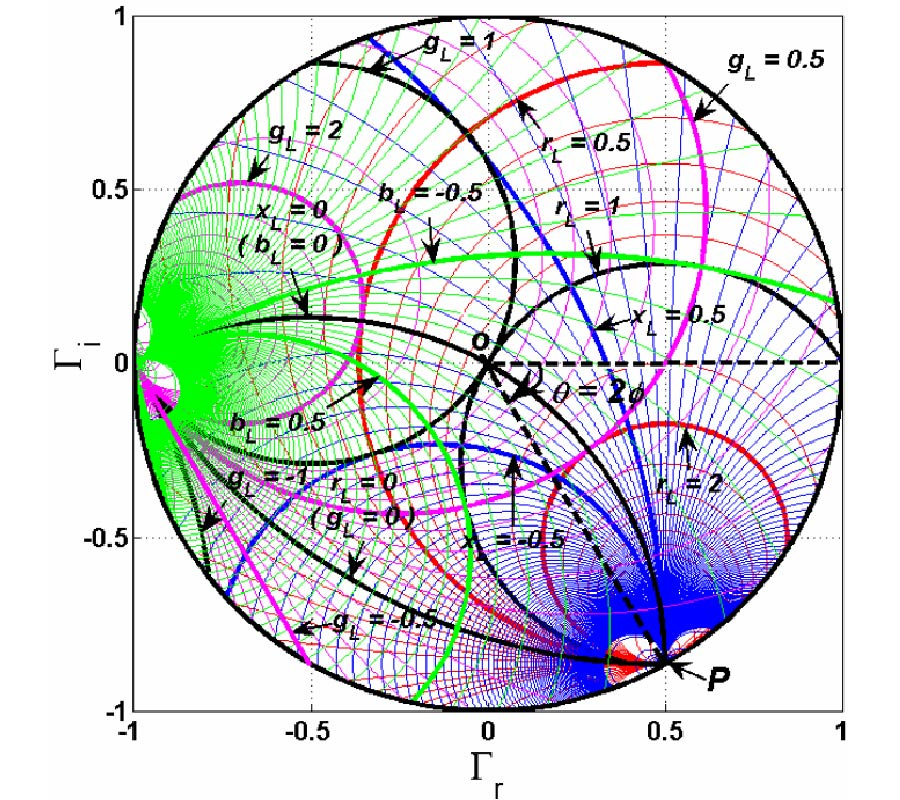2009-06-30
PIER M
Vol. 7, 179-192, 2009
Simultaneous TE and TM Surface Polaritons in a Bilayer Composed of a Single-Negative Materials
We investigate the dispersion properties of both TE and TM surface polariton modes formed at the surfaces of a bilayer composed of a single-negative materials. The dispersion curves of surface polaritons modes is found to consist of two branches, and it is shown that TE and TM surface polaritons may have a simultaneous mode. The characteristics of TE and TM surface polaritons modes (the frequency, localization position, ...) are shown to depend on the relative thicknesses of two single-negative layers of the bilayer. We find that the TE and TM surface polariton modes propagate in the same directions along the interfaces of the bilayer in the most cases. Nevertheless, the TE and TM surface polariton modes may have opposite directions of propagation for appropriate thicknesses of two single-negative layers. This can be interesting especially in the case of simultaneous TE and TM surface polariton mode, for which the structure acts as a polarizing beam splitter.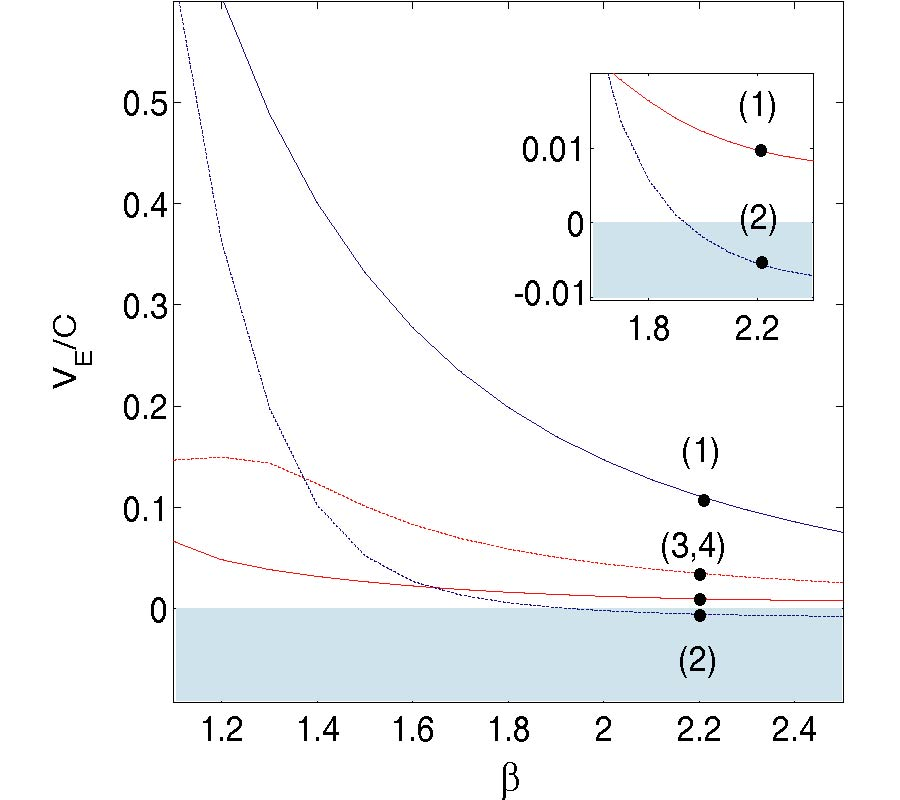2009-06-18
PIER M
Vol. 7, 165-177, 2009
A New Integral Equation Formulation for Scattering of Electromagnetic Waves by 2D Conducting Structures, Using Cylindrical Harmonics
Nima Chamanara
Using cylindrical harmonics and Fourier series, a new integral equation formulation is derived for perfectly conducting 2D scattering problems. This new integral equation is based on the fact that, all of the electric and magnetic field components are zero inside a perfect electric conductor. The incident and scattered fields are expressed in the cylindrical coordinate system with respect to a common origin inside the scatterer, using the addition theorem for Bessel and Hankel functions. The resulting electric or magnetic field is set equal to zero for all the points inside the largest cylinder that is contained in and tangent to the surface of the scatterer. As a result the field point variables are eliminated from the integral equation and only the source points are present in this formulation. Therefore the size of the problem is reduced considerably. A dramatic improvement in the computation speed is seen compared to the classical method of moments. TE and TM scattering problems are considered and the integral equation formulation is derived and solved for both cases.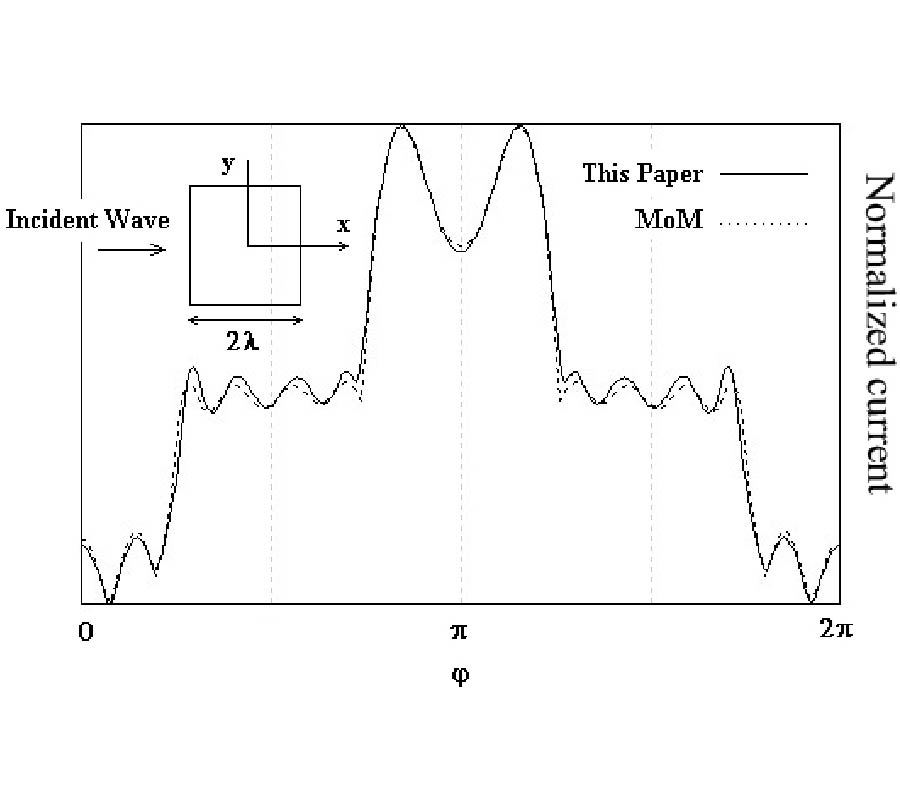2009-06-13
PIER M
Vol. 7, 149-164, 2009
Random Errors Modelling and Their Effects Upon RCS for an Artificial Object Containing Thin Long PEC Needles
Zeshan Akbar Awan and Azhar Abbas Rizvi
The scattering of electromagnetic plane wave from an artificial object containing thin long perfectly conducting needles embedded in a homogeneous background material is characterized by parameters like positioning, orientations and lengths of needles. Firstly, models of random errors in positioning and orientation of perfectly conducting needles are proposed. Secondly, their effects upon ensemble averaged RCS is analyzed. It is investigated theoretically that increasing error in positioning and orientation of conducting needles reduces ensemble averaged RCS.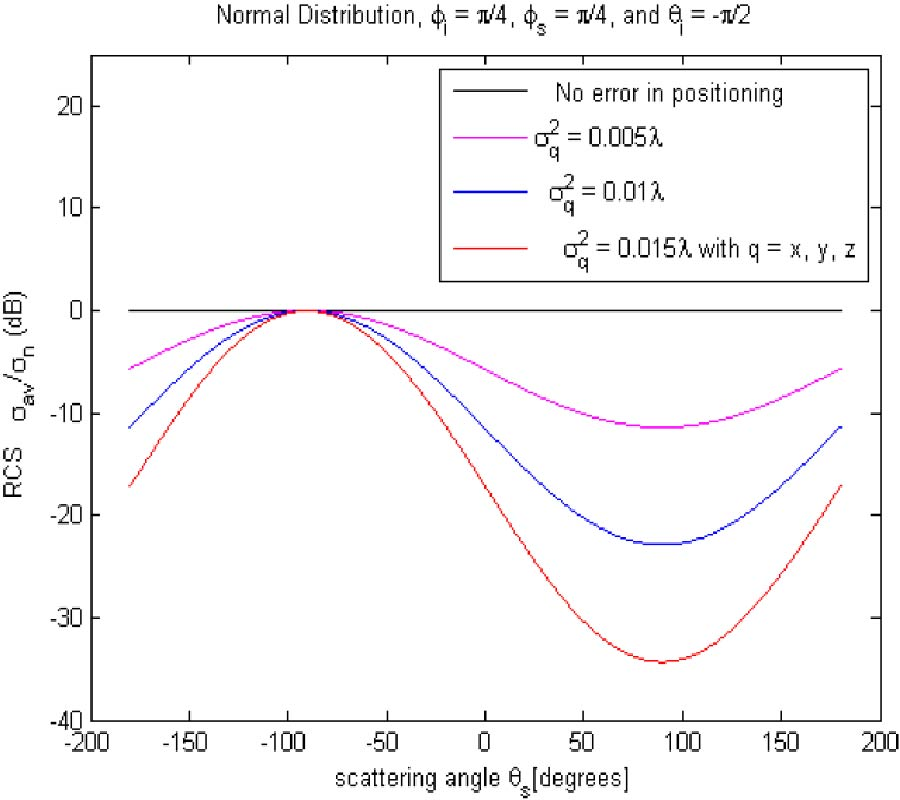2009-06-12
PIER M
Vol. 7, 135-148, 2009
Development and Application of an Enhanced MoM Scheme with Integrated Generalized n -Port Networks
Faik Bogdanov , Roman Jobava , Anna L. Gheonjian , Ekaterina A. Yavolovskaya , Natalia G. Bondarenko , Teimuraz N. Injgia and Stephan Frei
This paper proposes an enhanced MoM scheme to integrate arbitrary N-port networks into geometry models. This scheme is based on the incorporation of network equations into the standard MoM scheme. The suggested scheme is validated by comparison of the characteristics of a linear amplifier with those obtained by PSPICE. A general application of the enhanced MoM scheme is to handle complicated antenna or EMC problems including various types of network devices. Its potential to handle antenna-amplifier systems, automotive or other EMC problems is outlined.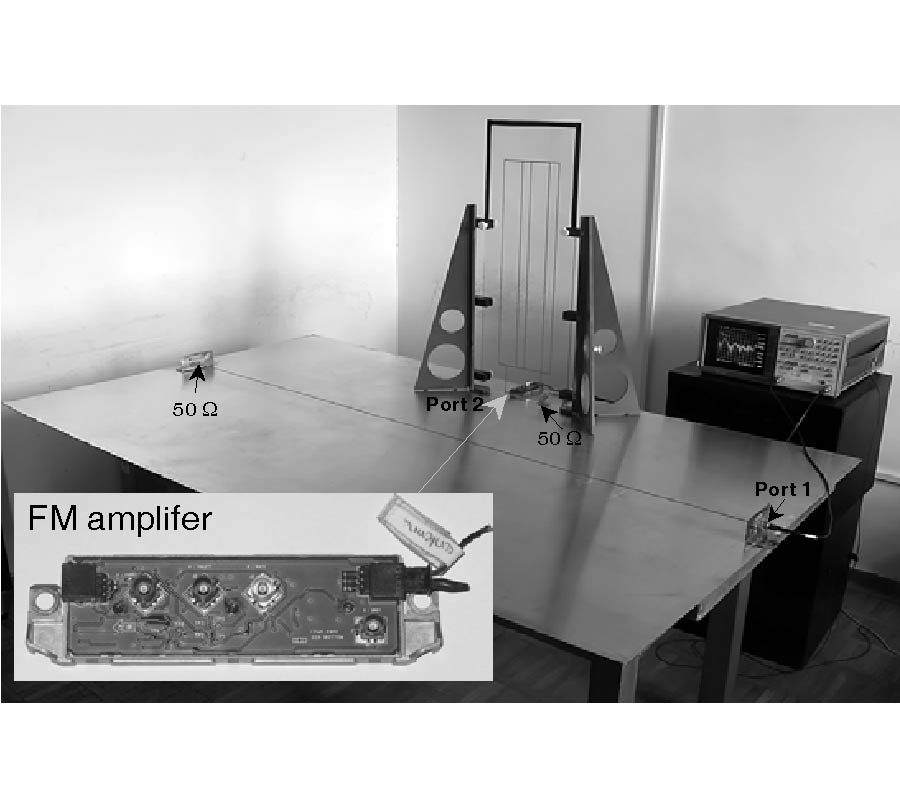2009-06-11
PIER M
Vol. 7, 123-133, 2009
Scattering from Perfectly Magnetic Conducting Surfaces: the Extended Theory of Boundary Diffraction Wave Approach
Ugur Yalcin
In this paper, the uniform scattered fields from a perfectly magnetic conducting (PMC) surface are studied with the extended theory of boundary diffraction wave (TBDW). The vector potential is described by considering the extended TBDW for the PMC surfaces. The extended TBDW is then applied to the problem of scattering from the PMC half plane. The total scattered fields are obtained and compared numerically with the exact solution for the same problem. The numerical results show that the solution of the extended TBDW is very close to the exact solution.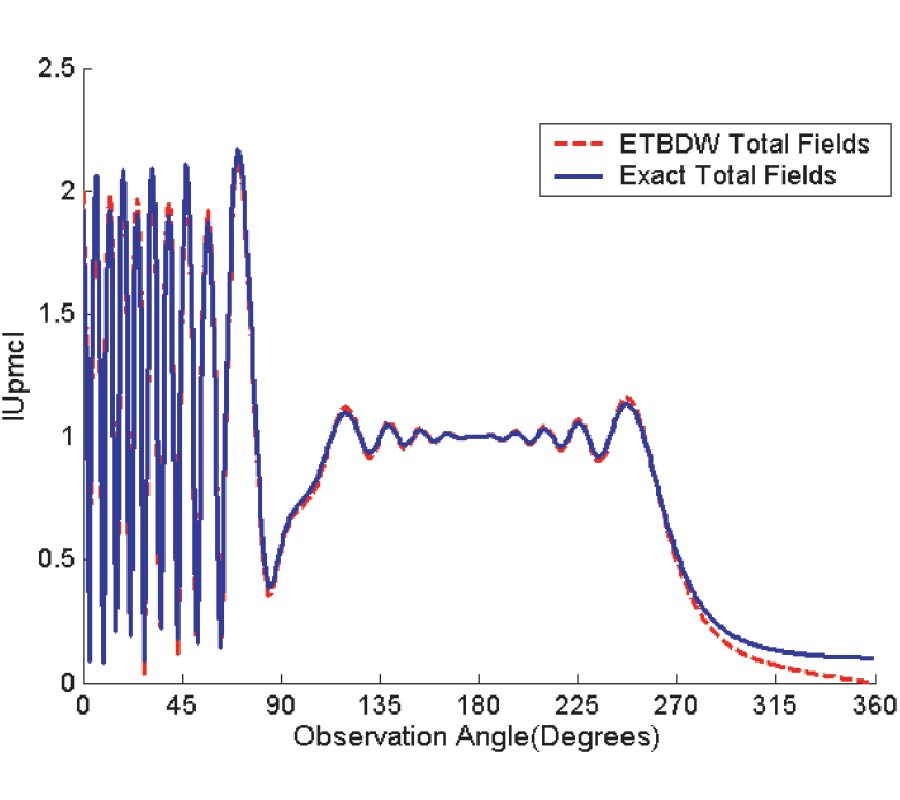2009-06-04
PIER M
Vol. 7, 109-122, 2009
A Simple Numerical Method to Compute the Signal-to-Noise Ratio of a Magnetic Resonance Imaging Surface Coil
Rafael Rojas Rodriguez and Alfredo O. Rodriguez Gonzalez
The quality of a magnetic resonance image can be reliably measured by the signal-to-noise ratio. This widely accepted parameter is a function of the magnetic field generated by the coil and the electric field produced by the sample to be imaged. A simple numerical method is proposed to calculate the coil signal-to-noise ratio of a circular-shaped coil and a spherical phantom. The phantom is composed of two-concentric sphere simulating a brain-skull model. The electromagnetic fields produced were then numerically computed by solving Maxwell's equations with the finite element method implemented in a commercial software tool. The electric and magnetic fields were used to numerically determine the signal-to-noise ratio using the quasi-static approach. The numerical results demonstrated that this simple method is able to calcualte the signal-to-noise ratio of surface coils with simple coil geometries involving a simulated phantom.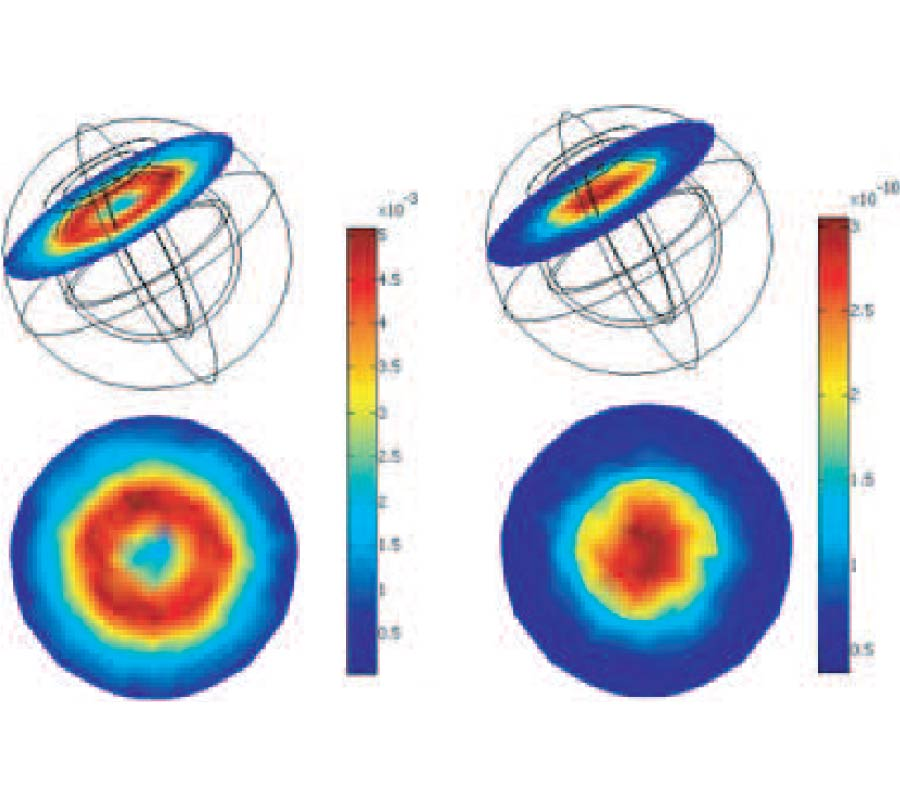2009-05-15
PIER M
Vol. 7, 97-108, 2009
Protection of Car-Size Sensitive Equipments Using a Shielding Cover
In this paper, the protection of a sensitive car-size system using a hemispherical shielding cover is discussed. The shield cover spreads on the soil, inserting some vertical (metal) rods into the ground, and adding a gridded wire carpet under the cover structure. The shielding performance of the cover structure was considered by a numerical simulation and experiments. The proposed cover structure shows the shielding effectiveness (SE) of -40 dB in worst case in the frequency range of 10 to 200 MHz.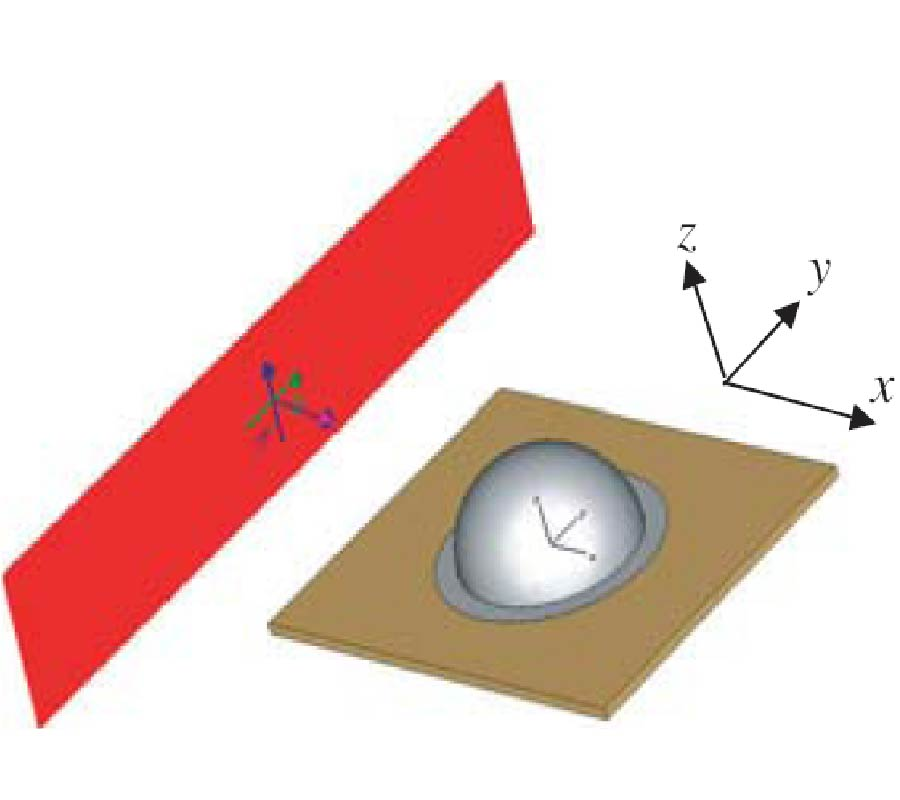2009-05-13
PIER M
Vol. 7, 87-96, 2009
A New Method for Determining the Scattering of Linear Polarized Element Arrays
Bao Lu , Shu-Xi Gong , Shuai Zhang and Jin Ling
A new method for analyzing the RCS of array antennas is presented in this paper. In the proposed method, the total RCS of the array can be simply decomposed to array RCS factor and element RCS factor. By this decomposition, the effects of the array distribution and antenna elements on scattering can be clearly exhibited. Thus, the analyzing of scattering characteristic of array antennas becomes easier. Moreover, proposed method has good compatibility for calculating the RCS of the array antennas with the same array distribution for different element.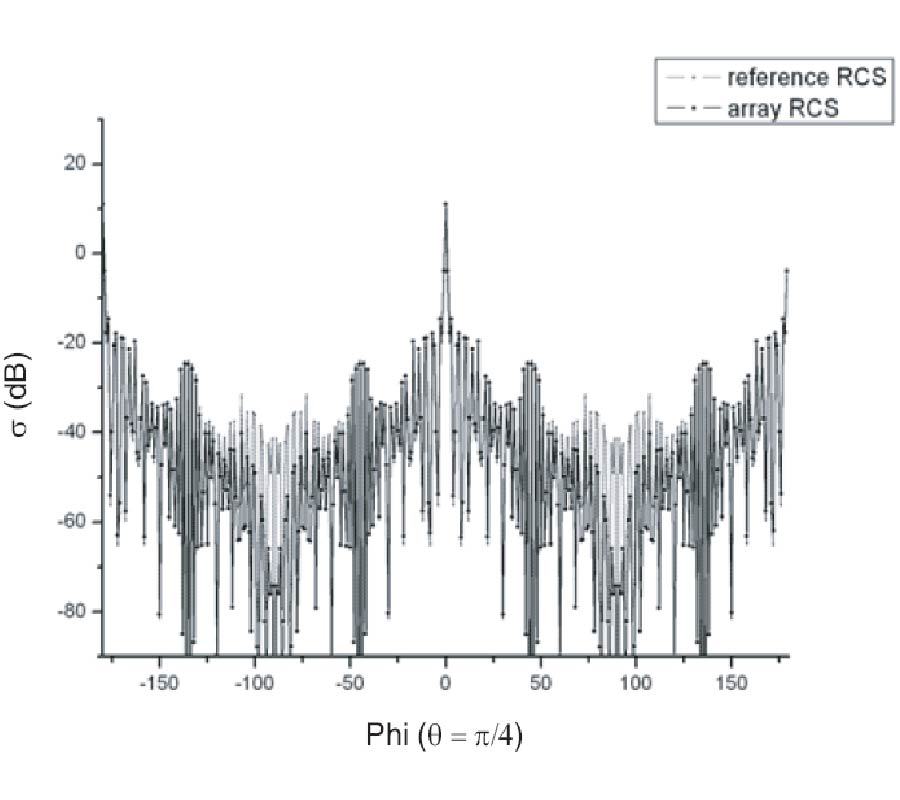2009-05-11
PIER M
Vol. 7, 71-85, 2009
Transmission Spectra in Ferrite-Semiconductor Periodic Structure
Oksana V. Shramkova
The specific features of TE-wave propagation in a structure fabricated by periodic alternating ferrite and semiconductor layers are investigated. Dispersion characteristics of eigenwaves are calculated. The transmission spectra of an electromagnetic wave oblique incident from the uniform medium onto the multilayer periodic ferrite-semiconductor structure is considered. The features of transmission spectra of the structure with periodicity breakdown are studied.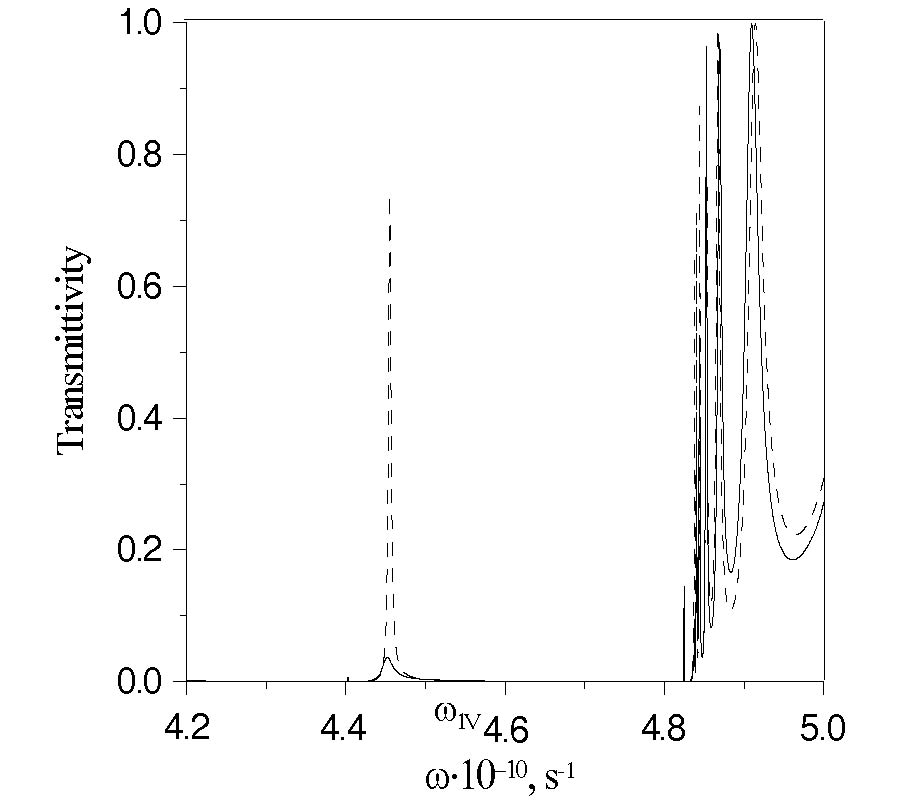2009-04-29
PIER M
Vol. 7, 57-69, 2009
A Synthesis of Unequally Spaced Antenna Arrays Using Legendre Functions
Jorge Sanchez , David H. Covarrubias-Rosales and Marco A. Panduro
In the wireless communication systems, the objective of using array antennas is to extract the desired signal while filtering out the unwanted interferences. New methods are developed for the optimization and synthesis, in terms of the directivity and the side lobe level. For the optimization of the array pattern, it is proposed to adjust both the excitation and the spacing of the antenna elements. The determination of the optimal excitation and spacing is shown to be a polynomial problem; this is described in terms of a unified mathematical approach to nonlinear optimization of multidimensional array geometries. The approach utilizes a class of limiting properties of Legendre functions that are dictated by the array geometry addressed. The objective of this paper is to describe a unified mathematical approach to nonlinear optimization of multidimensional array geometries.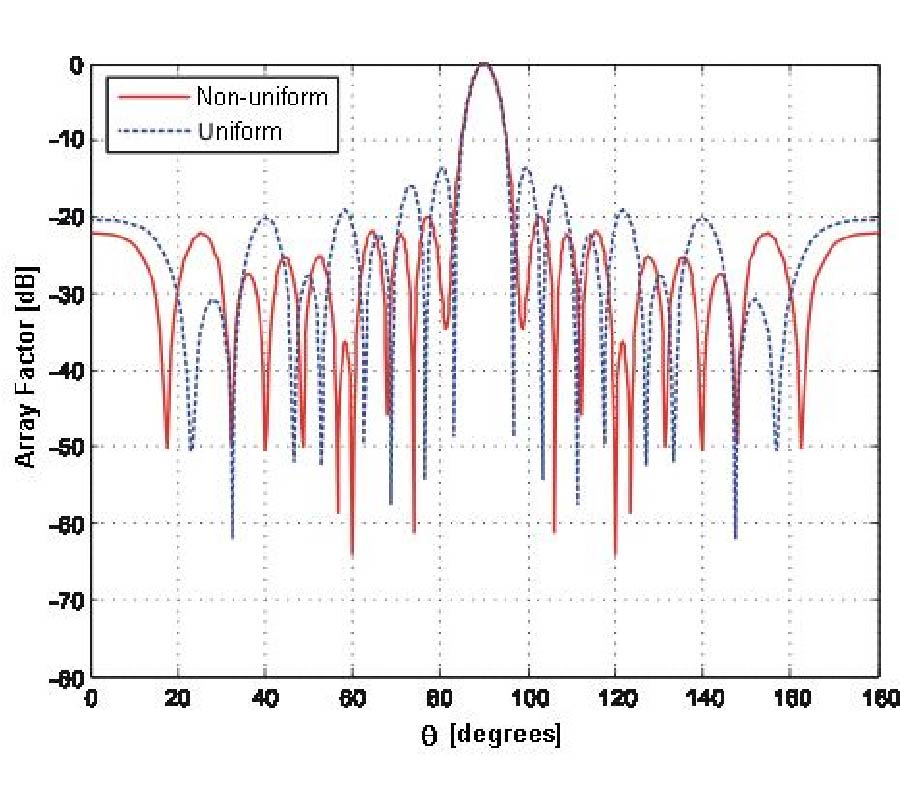2009-04-28
PIER M
Vol. 7, 41-55, 2009
Sandeepak S. Kakatkar and Kamla Prasan Ray
In this paper, the cosinusoidal slot aperture distribution is replaced with a two term approximation. Using this two term approximation, the slot fields are evaluated in closed form and explicit expressions are given in terms of sine and cosine integrals. The two term approximation and the near fields derived therefrom agree closely with the cosinusoidal distribution for slot lengths upto 0.65λ, with an error of less than 3.3% with respect to the numerical results upto 0.05λ from the slot in the near field. The formulation given here is of practical use in estimating mutual coupling in an array or in estimating radiated emissions for Electromagnetic Compatibility (EMC) analysis.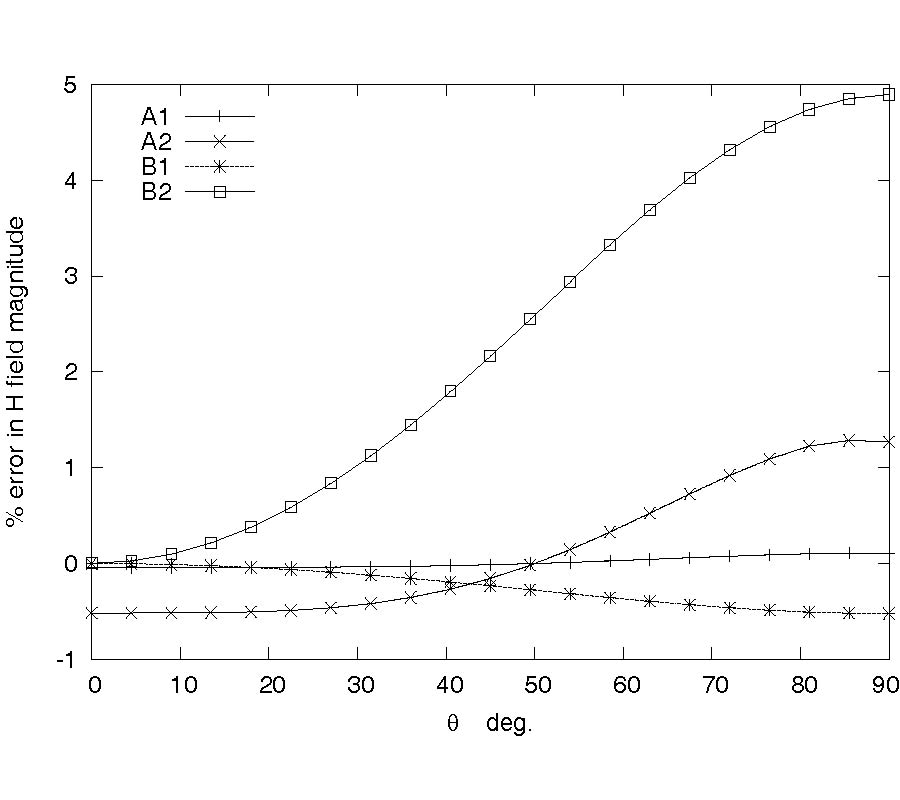2009-04-21
PIER M
Vol. 7, 29-39, 2009
Uniform Scattered Fields of the Extended Theory of Boundary Diffraction Wave for PEC Surfaces
Ugur Yalcin
In this paper, the uniform scattered fields from a perfectly conducting (PEC) half plane are studied with the extended theory of the boundary diffraction wave. A new vector potential of the boundary diffraction wave is found by considering the Fermat principle for the PEC surfaces. This vector potential is applied to the Helmholtz-Kirchhoff integral, and the theory of the boundary diffraction wave is extended to the PEC surfaces. The extended theory of the boundary diffraction wave is then applied to the scattering problem for the PEC half plane. The total scattered fields are compared numerically with the exact solution for the same problem. The numerical comparisons given in the paper show that the solution of the extended theory of the boundary diffraction wave is very close to the exact solution.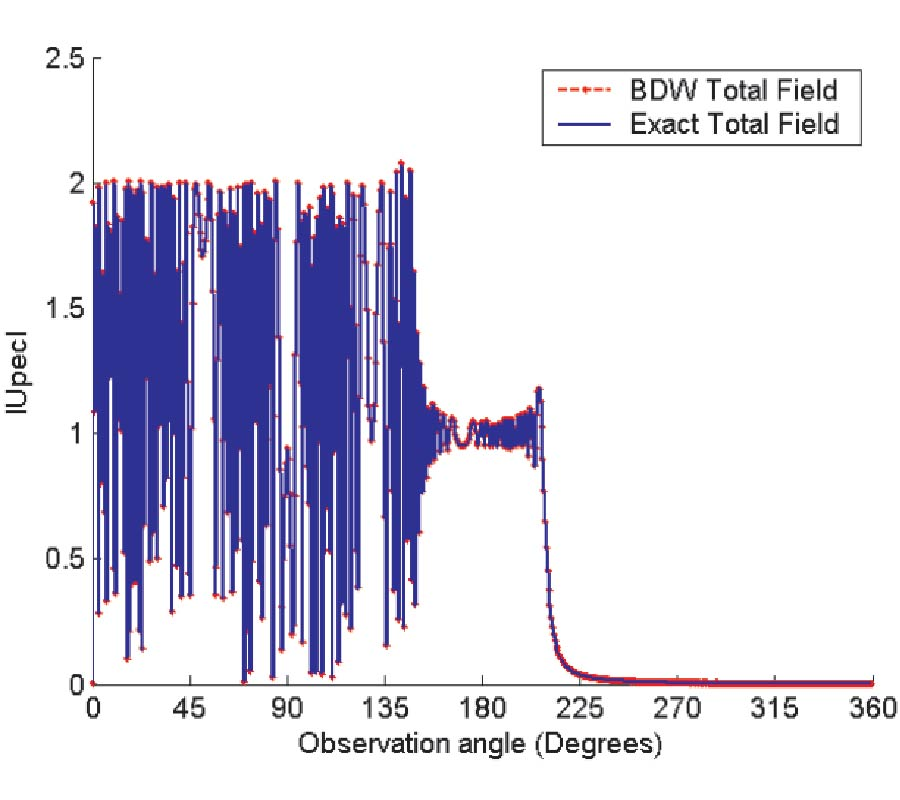2009-04-17
PIER M
Vol. 7, 15-28, 2009